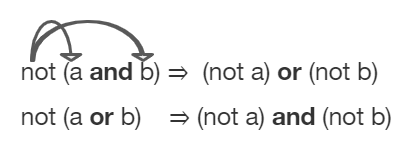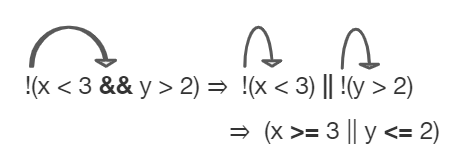# 3.6. Equivalent Boolean Expressions (De Morgan’s Laws)¶

What if you heard a rumor about a senior at your school? And then you heard that the rumor wasn’t true. Which part of “a senior at your school” wasn’t true? Maybe they weren’t a senior? Or maybe they didn’t go to your school? You could write this as a logic statement like below using negation (!) and the AND (&&) operator since both parts have to be true for the whole statement to be true. (Thank you to Kevin Saxton from Kent School, CT for this example.)

```!(a && b)

a = "senior"
b = "at our school"
```

In this lesson, you will learn about De Morgan’s Laws which simplify statements like this. We know that !(a senior at our school) could mean !(a senior) or !(at our school). Let’s learn more about De Morgan’s Laws.

De Morgan’s Laws were developed by Augustus De Morgan in the 1800s. They show how to simplify the negation of a complex boolean expression, which is when there are multiple expressions joined by an AND (&&) or OR (||), such as `(x < 3) && (y > 2)`. When you negate one of these complex expressions, you can simplify it by flipping the operators and end up with an equivalent expression. De Morgan’s Laws state the following equivalencies. Here’s an easy way to remember De Morgan’s Laws: move the NOT inside, AND becomes OR and move the NOT inside, OR becomes AND.Figure 1: De Morgan’s Laws to simplify complex expressions

In Java, De Morgan’s Laws are written with the following operators:

• !(a && b) is equivalent to !a || !b

• !(a || b) is equivalent to !a && !b

Going back to our example above, !(a senior && at our school) is equivalent to !(a senior) or !(at our school) using De Morgan’s Laws:

```!(a && b) is equivalent to !a || !b

a = "senior"
b = "at our school"
```

You can also simplify negated boolean expressions that have relational operators like <, >, ==. You can remove negation by moving it inside and flipping the relational operator to its opposite sign. For example, not (c equals d) is the same as saying c does not equal d. An easy way to remember this is Move the NOT inside, flip the sign (== becomes !=, < becomes >=, and > becomes <=).

• !(c == d) is equivalent to (c != d)

• !(c != d) is equivalent to (c == d)

• !(c < d) is equivalent to (c >= d)

• !(c > d) is equivalent to (c <= d)

• !(c <= d) is equivalent to (c > d)

• !(c >= d) is equivalent to (c < d)

You should be able to show that two boolean expressions are equivalent. One way to do this is by using truth tables. For example, we can show that !(a && b) == !a || !b by constructing the truth table below and seeing that they give identical results for the 2 expressions (the last 2 columns in the table below are identical!).

a

b

!(a && b)

!a || !b

true

true

false

false

false

true

true

true

true

false

true

true

false

false

true

true

Often, you can simplify boolean expressions to create equivalent expressions. For example, applying De Morgan’s Laws to `!(x < 3 && y > 2)` yields `!(x < 3) || !(y > 2)` as seen in the figure below. This can then be simplified further by moving the not operator inside and flipping the relation operators. So, `!(x < 3) || !(y > 2)` is simplified to `(x >= 3 || y <= 2)` where the relational operators are flipped and the negation is removed. These two simplification steps are seen below.Figure 2: An example boolean expression simplified

For what values of x and y will the code below print true? Try out different values of x and y to check your answer.Check your understanding

## 3.6.1.Programming Challenge : Truth Tables¶

Explore the following problems. You may use this worksheet to complete your truth tables. Assume that x is an integer value, for example -1, 0, or 1.

1. Complete a truth table for the boolean expression: !(x == 0 || x >= 1). Is this the set of positive or negative numbers? Is the expression true when x is positive? Or is it true when x is negative? You can try out the values when x is 1 or -1 or 0. Note that 0 is not positive or negative. You can try running the code below to check your answer.

2. Complete a truth table for the boolean expression: !(x == 0) && !(x >= 1). Is this the set of positive or negative numbers?

3. Complete a truth table for the boolean expression: (x != 0) && (x < 1). Is this the set of positive or negative numbers?

4. Are the 3 boolean expressions equivalent? Why or why not?

Are these 3 boolean expressions equivalent? 1. !(x == 0 || x >= 1) , 2. !(x == 0) && !(x >= 1) , 3. (x != 0) && (x < 1)

## 3.6.2. Summary¶

• De Morgan’s Laws can be applied to Boolean expressions to create equivalent ones:

• !(a && b) is equivalent to !a || !b

• !(a || b) is equivalent to !a && !b

• A negated expression with a relational operator can be simplified by flipping the relational operator and removing the not.

• !(c == d) is equivalent to (c != d)

• !(c != d) is equivalent to (c == d)

• !(c < d) is equivalent to (c >= d)

• !(c > d) is equivalent to (c <= d)

• !(c <= d) is equivalent to (c > d)

• !(c >= d) is equivalent to (c < d)

• Truth tables can be used to prove that 2 Boolean expressions are identical.

• Equivalent Boolean expressions will evaluate to the same value in all cases.